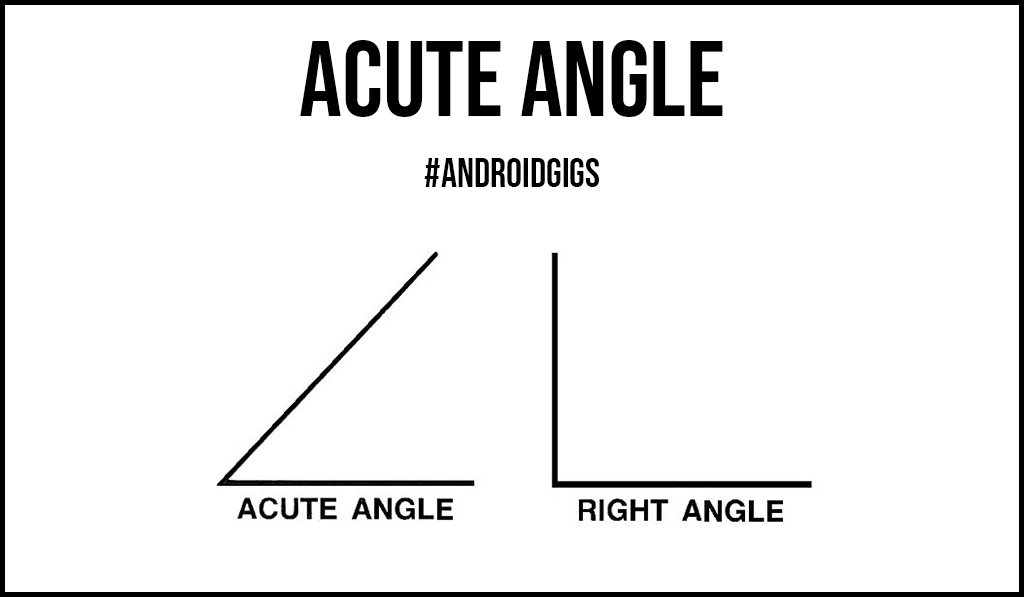# All You Need To Know About Acute Angle

Have you observed the hands of a clock when it shows 11:00 A.M. or the pairs of scissors?

All these objects or real-life scenarios show the acute angle. An acute angle is an angle that can be defined as an angle that measures less than 90 degrees.

Some examples of an acute angle are as follows: 50 degrees, 75 degrees, 80 degrees, 65 degrees, 35 degrees, and so on.

Other than acute angle, there are various other types of angles such as obtuse angle, supplementary and complementary angle, right angle, and many more.In this article, we will try to cover some basic aspects about the types of angles like; properties of an acute angle, real-life examples of it, and do a detailed analysis about these topics.

## Some Significant Properties of Acute Angle

An acute angle is an angle that measures less than 90 degrees. The following points analyze the important properties of acute angle:

1. The angles always measure less than 90 degrees such as 50 degrees, 75 degrees, 80 degrees, 65 degrees, 35 degrees, and so on.
2. The acute angles are classified into various types on the basis of their nature such as acute scalene, acute isosceles, and equilateral triangle.
3. An equilateral triangle is a type of triangle where every angle measures 60 degrees resulting in the formation of 180 degrees. (60 + 60 + 60 = 180).
4. Other than acute angles, there are various types of angles that are classified on the basis of degrees. Some of them are right angle, obtuse angle, straight angle, and many others.

## Real-life examples of Acute Angles

The following points analyze the real-life examples which will help to identify or determine the acute angles more easily:

1. A watermelon when cut into four pieces forms an acute angle (less than 90 degrees).
2. The hands of a clock when it shows 11:00 A.M is an example of an acute angle. There are other instances as well where the clock hands on the minute sides form an acute angle.
3. There are various types of birds which consist of a beak. All these beaks can be regarded as acute angles.
4. You might have not seen a crocodile in real life, if the mouth of a crocodile is open, it can be considered as an example of an acute angle.

## Acute Angle Vs Obtuse Angle Vs Right Angle

In the next few points, you may see some points which distinguish one angle from another. Some of the points are mentioned below:

1. An obtuse angle is an angle that measures less than 180 degrees and more than 90 degrees. It is also known as ‘stupid angle’. A right angle is a type of angle that exactly measures 90 degrees. An acute angle is an angle that can be defined as an angle that measures less than 90 degrees.
2. Some examples of obtuse, right, and acute are given below respectively. 120 degrees, 130 degrees, 95 degrees. Right angle = 90 degrees.  50 degrees, 75 degrees, 80 degrees.